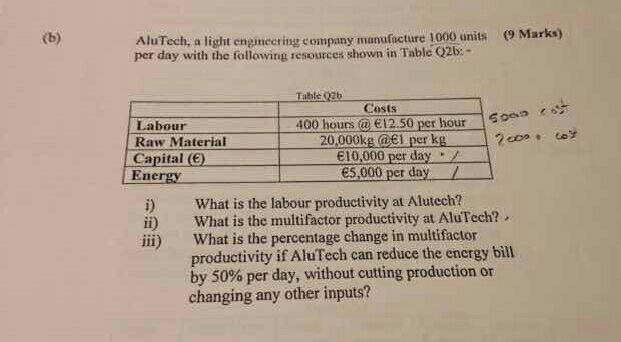# Question & Answer: Alu Tech, a legit engineering company manufacture 1000 units per day with the following resource…

Alu Tech, a legit engineering company manufacture 1000 units per day with the following resource shown in Table Q2b: What is the labor productivity at Clutch? What is the multifactor productivity at Ale Tech? What is the percentage change in multi factor productivity if Ale Tech can reduce the energy bill by 50% per day, without cutting production or changing any other inputs?a) Labor Productivity

Labor Productivity is generally defined in terms of amount of goods and services produced by one hour of labor

Don't use plagiarized sources. Get Your Custom Essay on
Question & Answer: Alu Tech, a legit engineering company manufacture 1000 units per day with the following resource…
GET AN ESSAY WRITTEN FOR YOU FROM AS LOW AS \$13/PAGE

Labor Productivity = Units produced / Labor hours = 1000 / 400 = 2.5 units/hr

b) Multifactor Productivity

Multifactor Productivity is defined as the ratio of output goods and/or services produced to all the costs involved

Multifactor Productivity = Output / (Raw material + Labor + Electricity + Capital)

Here, Raw material costs = 20000 * 1 = 20000

Labor costs = 12.5 * 400 = 5000

Energy costs = 5000

Capital costs = 10000

Multifactor Productivity = 1000 units / (20000 + 5000 + 5000 + 10000) = 1000 / 40000 = 0.025 units/\$

c) Change in Multifactor Productivity %

If energy costs is reduced by 50%, the new energy cost will become 2500.

So, Multifactor Productivity = 1000 units / (20000 + 5000 + 2500 + 9000) = 1000 / 36500 = 0.0274 units/\$

Change in Multifactor Productivity % = (0.0274 – 0.025)/ 0.025 * 100 = 9.6%